# 13 Best time calculator apps for Android & iOS

Sometimes we need to make complex calculations related to time. With the help of a regular calculator, it is difficult to calculate hours, minutes and seconds. For this purpose, special applications have been created which greatly simplify this process.

This list contains the best time calculator apps. They will help you to make accurate calculations.

## Time Calculator: Hours Work & Time BetweenThe Time Calculator: Hours Work & Time Between time calculator allows you to perform many calculations. They save time for modern people and allow them to keep up with it.

The application window is like a desktop calculator. It has extra buttons – “Year”, “Day”, “Hour”, “Min, “Sec”. These are the buttons that make it possible to calculate the time.

Besides, the application Time Calculator: Hours Work & Time Between also has a virtual button “Convert”. It allows you to convert time values from one unit to another.

When you touch the “Convert” button, you go to the window where you can see how many years, days, hours, minutes and seconds the entered period is.

This does not exhaust the possibilities of the time calculator under consideration. It allows you to perform actions over time values. All calculations made by this app are performed in the same way as in a normal calculator.

It is more difficult to perform calculations with dates. Because leap years are not taken into account. This factor will have to be calculated in your mind anyway.

At the same time, it is very convenient to make calculations within one day.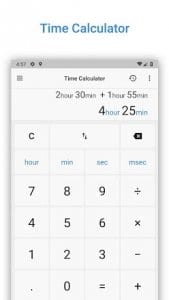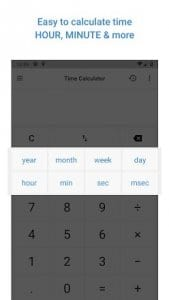This application also performs calculations over ordinary numbers.

You may also like: 20 Best Calculator apps for iPhone & Android

## Time CalculatorTime Calculator is a professional time calculator. Also in this app, there is a calculator for regular calculations.

This application can perform various mathematical calculations. It can also convert time units into other ones. This application is useful for those for whom time tracking is an integral part of life.

This time calculator can calculate years, days, hours, minutes and seconds. You can also combine different time units. Some time units can be converted to others.

So, you don’t have to make complex computational processes on your own. The application Time Calculator will do it for you.

The formulas you use to calculate time can be saved. They can be used later on to avoid entering values again. You can also use this application as a regular calculator. This is very convenient and practical.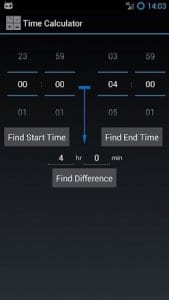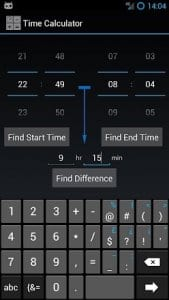It is very easy to use this calculator. First, select a value. Next, enter the number. Repeat these steps until you enter the last value you want. By clicking on the “Conversion” button, you can convert the entered value to a certain unit of time.## Time and Hours CalculatorTime and Hours Calculator is an excellent tool for calculating time values.

If you often work with dates and times, this application will be a great help for you. It makes it easy for you to plan your future actions and events.

This application will help you calculate the duration between two dates or times. Thus, you can calculate the total number of years, months, days, hours, minutes and even seconds.

You can use it to calculate the time between important dates in your life. The calculation process in this app is very simple and clear.

A wide variety of computational actions are available here. You can add up two dates or two times and subtract them. It is also useful for finding the leap year and the amount of time left before it.

You can also find out how many specific days of the week are left before the date you selected. For example, find out how many days off you have left before your next paycheck.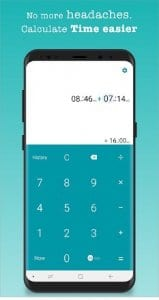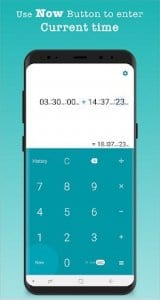You can also calculate the number of working days and days off in a certain period of time.Add Hours and Minutes allows you to quickly and easily calculate the amount of time you need.

You can use this application whenever and wherever you want. This is especially useful for people whose work involves measuring time.

There are many different actions available in this application. Add and subtract dates and times. You can connect different time units to each other. For example, hours and seconds.

All mathematical calculations and conversion will be done for you. All your calculations are saved in the history of the application Add Hours and Minutes. Also, this calculator is able to work with decimal indices.

You can even know your age in days, hours, minutes or seconds. Turn on the countdown to know how many days or hours are left until your selected date.

You can also use this calculator to calculate your financial savings and contributions. In the special tab of the application Add Hours and Minutes, you can find information about time zones and world time.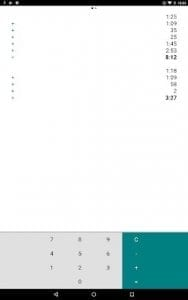This is a free and easy to use app that runs without restrictions and does not need built-in shopping.## Time CalcThe Time Calc app can calculate the time interval between two specific indicators.

You can choose from a range of time indicators such as year, month, week, day, hour, minute and second. As you can see, this calculator is multifunctional.

It is capable of making the most accurate calculations. Various computational actions are available here. You can add and subtract dates.

Find out how many working days are left before your next weekend off. You can also use this calculator to find out how many days of the week you have selected to a certain date.

Use this app to surprise your friends. Find out their age in minutes or even seconds. You can save the result and then send it by mail or social media.

You can also use this calculator for normal computing. You can use it even in stores while shopping.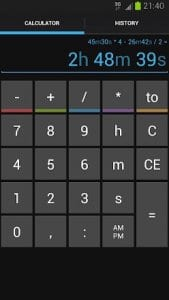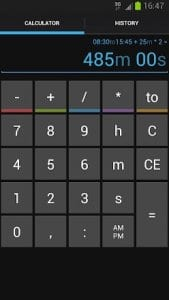This free application supports 12-hour and 24-hour time formats. Use the one that suits you. This way, the calculations become even easier and more accessible.

## Time Calculator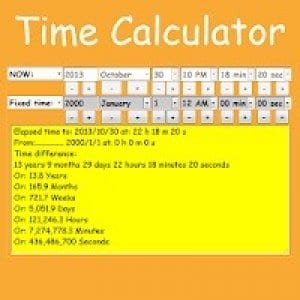Time Calculator is an easy-to-use time calculator with rich functionality.

This application allows you to calculate the time interval between the dates. Simply enter two values and start the calculation process.

You will almost get the ready-made time parameters in days, hours, minutes and seconds.

It is very convenient to use to calculate the time you are at work. So, when you come to work, click on the “Start” button, which is located on the main screen of the application Time Calculator.

When your working day is over, click on the “Stop” button. This is how you commit the end of your work shift. Now you can get the result. It shows how many hours, minutes and seconds you have spent at work.

The finished report can be sent to your supervisor, for example, by email. To do this, you can enter your contact details on a special menu in the application settings.

Also thanks to this application you can calculate your salary. Simply enter the required data and click “Calculate”.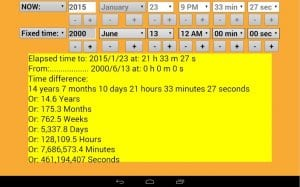As you can see, the application Time Calculator can be used for a variety of purposes.## Time CalculatorThe Time Calculator app can make any mathematical manipulations with dates and times. It is suitable for calculating the number of days, hours, minutes and seconds.

It can be useful for quick calculations if you don’t have a calendar at your fingertips. Or if you just don’t want to calculate everything yourself.

So, with the help of this application, you will know your age in days, hours, minutes and even seconds. You will also be able to calculate the amount of time left until an important date.

These are just a few examples of when you can use this application. It is definitely useful for you always and everywhere.

Calculating the length of time between two indicators, you can find out its middle. The app Time Calculator also indicates the time before and after the date you specify, if necessary.

This feature should be enabled in the application settings. You can add more days from the date you have selected, as well as more days.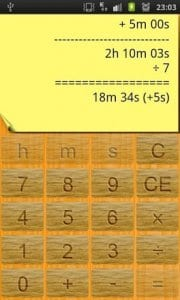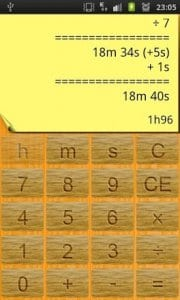You can also view the days of the week when calculating the number of days. If you are interested in the specific days of the week, you can exclude unnecessary days. For example, this will be useful for calculating the number of days off in the period of time you have selected.

## Fast Time CalculatorThe Fast Time Calculator app can make accurate mathematical calculations without much difficulty.

Enter the desired dates, subtract and add them. Also here you can add up various time figures. The application Fast Time Calculator can automatically convert temporary data.

So, if you need to know how many seconds in 32 hours, you can enter this indicator and quickly get the desired result.

Thanks to this application, you can calculate your age to the nearest second. If you want to know how many days are left to the important date, you can set the time count.

For example, the app Fast Time Calculator will let you know when there are only a few days or hours left until your selected date. This option is up to you.

This calculator is especially popular among pregnant women. So, you can calculate the expected date of birth or the number of days remaining.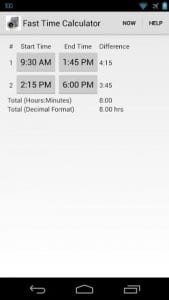You will also find out how long you are pregnant. Or even in which trimester is your pregnancy at the moment. This distinguishes this calculator from other similar applications.## Time CalculatorThe Time Calculator app is an excellent calculator for calculating the amount of time between two periods.

Use different mathematical operations. You can calculate and add times. The application can also convert numeric expressions. For example, you can convert a clock to seconds and much more.

Calculate how many days are left until an important date. Select the date you want from the list. Set a reminder in this app.

Find out what your age is. This feature allows you to calculate your age in days, hours, minutes and even seconds. Thanks to this, you can surprise your friends by calculating their rates.This free application has rich functionality and a nice interface. It differs from other analogs by its laconic design. Besides, it is very convenient to use wherever and whenever you want.

## Time Calculator Timesheet, Hours & Minutes Between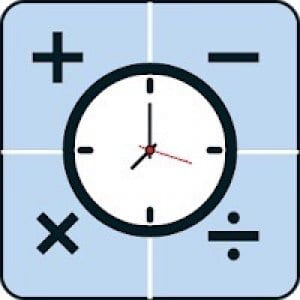Use the handy Time Calculator Timesheet, Hours & Minutes Between application for various time calculations.

Many different functions are available here. You can enter a date or time from the past and add the current time.

This will give you how much time has passed since the selected date to date. The result can be seen in hours, minutes or even seconds.

If you accidentally make a mistake when you enter a value, it is very easy to correct it. There is a button to cancel, which removes the last actions you have taken.

All your calculations are stored in the history of the application Time Calculator Timesheet, Hours & Minutes Between.

So, if you need to recalculate the period, you don’t have to re-enter the data at all. You can use the application’s Memories. Thus, it will automatically enter the values you have selected.

You can also delete unnecessary data in the application history. The finished result can be exported by sending it by e-mail or messenger.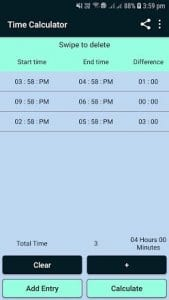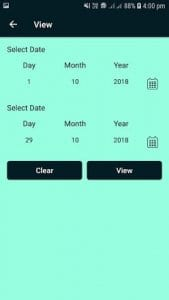This is convenient for employees who have calculated the time spent at work. You can send this figure to your supervisor with just a few clicks.## Time Calc – Date Time & Duration Calculator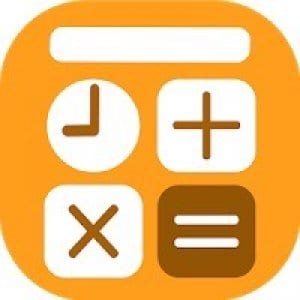The Time Calc – Date Time & Duration Calculator application is another handy tool that allows you to calculate the period.

It easily converts the data you specify. You do not need to count how many seconds in 72 hours. The app Time Calc – Date Time & Duration Calculator will do this for you if necessary.

This program can use days, hours, minutes and seconds in its calculations. You can also choose longer intervals.

For example, years, months and weeks. You can even enter specific dates and find out how many seconds between them.

This application has very rich functionality. Even the most complex calculations can be made here. So, you can enter two-time intervals. So, you will get information about the time between these intervals. Not every application can calculate such time periods.

Calculation results can be saved in the application library. They can also be shared via email or any social network account.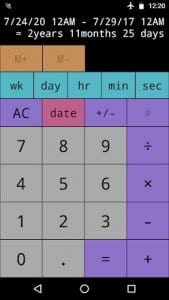This free app is sure to be useful for those for whom time is a valuable and vital resource.## Hours CalculatorHours Calculator is a simple application for fast and accurate time calculation.

It is able to automatically recognize which value you have entered. For example, you enter the numbers 24:60:30 and the app will highlight the hours, minutes, and seconds from this.

If the app Hours Calculator has made a mistake, you can adjust the values selected by the app. But, there are very few such cases, as the app works quite accurately and accurately.

It uses a special smart algorithm that can perform complex calculations. So, if you need to calculate a fairly long period of time, the application will do it without much difficulty.

Just enter all the parameters and you will get the result. You can even enter data from the past, such as in past years or months. Then select the current day or time.

For example, the app Hours Calculator will show you how much time has passed since the date you selected. This can be converted to hours, minutes and even seconds.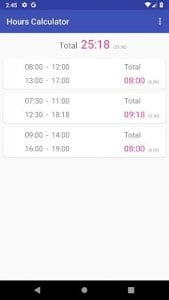This app is completely free. It does not contain any built-in advertising and does not need built-in purchases. Use this calculator without any restrictions.

## Time Calculator TrialThe Time Calculator Trial application can also perform complex time calculations to the nearest second.

It will open up new possibilities for your phone. It is very easy to use. The application works like a regular calculator but has many different interesting features.

The first time you start the app Time Calculator Trial, you will see the main crane, where you will perform all the calculations. There are separate fields for entering each value. You can enter two values.

Once you have entered the data, you need to choose what action the application should take. This can be adding or subtracting.

When adding up, you will see the total amount that can be expressed in any timeframe. When you subtract, you will see the difference between the time periods you have selected.

You will also be able to calculate how many days are left from today to the important event in your life.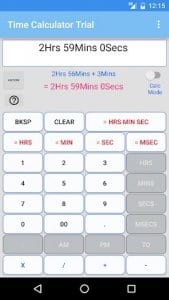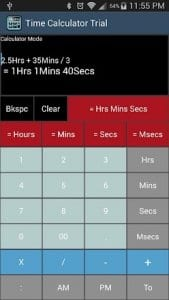This free application works without any restrictions. It does not need built-in shopping and has no annoying advertising. You can also use it as a multifunctional calculator for regular calculations.

You may also like: 12 Best apps for calculating sunrise and sunset times (Android & iOS)